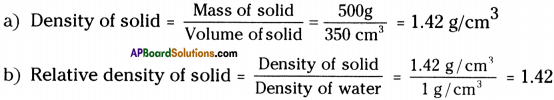# AP Board 9th Class Physical Science Important Questions Chapter 9 Floating Bodies

AP State Syllabus AP Board 9th Class Physical Science Important Questions Chapter 9 Floating Bodies

## AP State Syllabus 9th Class Physical Science Important Questions 9th Lesson Floating Bodies

### 9th Class Physical Science 9th Lesson Floating Bodies 1 Mark Important Questions and Answers

Question 1.
Scientifically what is density?
Density is defined as mass per unit volume.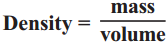Question 2.
Define relative density.
Relative density is the ratio of density of an object to the density of water.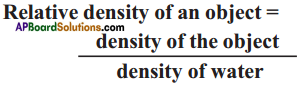Question 3.
What instrument is used to determine the purity of milk?
Purity of milk can be determined by using lactometer.

Question 4.
What instrument is used to know the relative density of liquids?
Relative density of liquids can be determined by using hydrometer.Question 5.
What is atmospheric pressure?
Air in our surroundings exerts pressure on the surface of earth called atmospheric pressure.
Atmospheric pressure P0 = ρhg

Question 6.
State Archimedes’ principle.
Archimedes’ principle states that when a body is immersed in a fluid it experiences an upward force of buoyancy equal to the weight of fluid displaced by the immersed portion of the body.

Question 7.
State Pascal’s principle.
Pascal’s principle states that external pressure applied to an enclosed body of fluid is transmitted equally in all directions throughout the fluid.

Question 8.
What is the principle involved in working of hydraulic lift?
Hydraulic lift works using Pascal’s law.Question 9.
In what direction does the buoyant force on an object immersed in a liquid act?
Buoyant force on an object acts upward direction.

### 9th Class Physical Science 8th Lesson Gravitation 2 Marks Important Questions and Answers

Question 1.
Define density and give its units.
Density is defined as the mass per unit volume.Units : Unit for density is gm/cm³ (or) kg/m³.

Question 2.
Define relative density and give its units.
Relative density is the ratio of density of an object to the density of water.Units : Relative density has no units.

Question 3.
Why does the wooden block float on water even though it is heavier than a marble?
The relative density of wooden block is less than the relative density of water whereas relative density of marble (glass) is more than that of water. Hence wooden block floats on water and marble sinks in water.Question 4.
What do we mean by ‘heavy’, what do we mean by ‘light’?
‘Heavy’ and ‘light’ can be decided based on the density of the object. Among the objects of equal volume, the one which weighs more can be treated as ‘heavy’ and the other one can be treated as ‘light’.

Question 5.
Relative density of silver is 10.8. What is density of silver in S.I. unit?
Relative density of silver = 10.8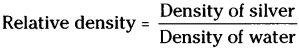Density of water in S.I. system = 0³ kg/m³.
Density of silver = Relative density × Density of water = 10.8 × 10³ kg/m³.

Question 6.
Why does a block of plastic released under come up to the surface of water?
Density of plastic is less than water. As the density of water is more than plastic, water exerts upward force which is known as buoyant force. So, the block of plastic come up to the surface of water.

Question 7.
The volume of 50 g. of a substance is 20 cm³. If the density of water is 1 g/cm³, will the substance float or sink?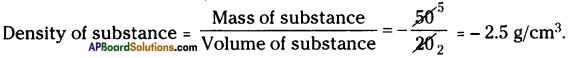The density of substance is more than water so the substance sinks in the water.

Question 8.
An iron nail sinks in water. While a large ship floats on the surface of water. Give reason.
The weight of water displaced by iron needle is less than its own weight, so it sinks. On the other hand, the ship displaces water more than its own weight, so it floats on water.

Question 9.
The density of gold is 19 g/cm³. Find the volume of 95 g of fold.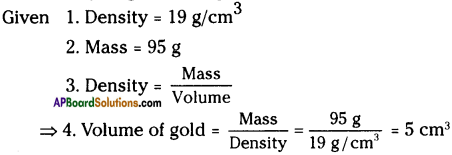Question 10.
A floating boat displaces water weighing 6000 newtons.
a) What is the buoyant force on the boat?
b) What is the weight of the boat?
a) By Archimedes’ principles, the buoyant force acting on the object by it.
So, the buoyant force on the boat = 6000 newtons.

b) According to the principle of floating
Weight of object = Weight of liquid displaced by it
⇒ Weight of the boat = 6000 newtonsQuestion 11.
The volume of 50 g. of a substance is 20 cm . If the density of water is 1 g cm-3, will the substance float or sink?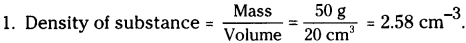2. Density of water = 1 g cm-3.
As the density of substance is greater than that of water, so, the substance will sink in water.

Question 12.
Write any two differences between density and relative density.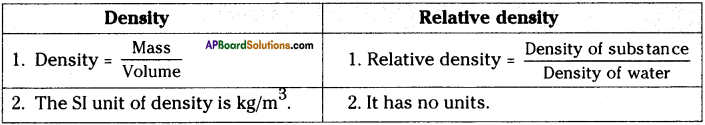### 9th Class Physical Science 8th Lesson Gravitation 4 Marks Important Questions and Answers

Question 1.
Derive an expression for atmospheric pressure.
1) All objects on the surface of the earth are subject to constant atmospheric pressure.

2) We know that the pressure is thrust (force) per unit area.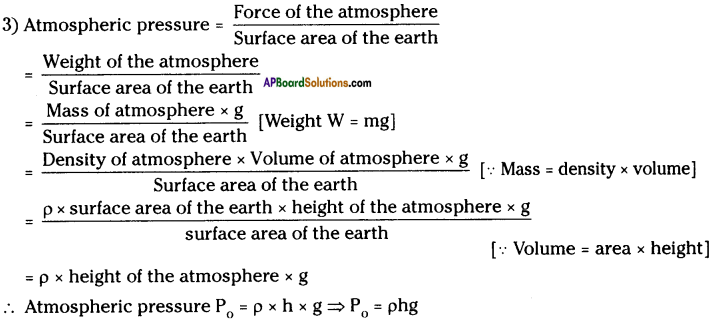Question 2.
How can you measure the atmospheric pressure?
1) Atmospheric pressure can be calculated with the help of the height of mercury column.

2) The weight of the mercury column in the tube is equal to the force applied on it by the mercury in the bowl due to atmospheric pressure.
∴ Weight of mercury column (W)
= Mass of mercury (m) × g
= Volume × density × g
= Cross sectional area of the tube × height of the tube × density × g
= Ahρg

Let P0 be the atmospheric pressure.
Force on the column due to atmospheric pressure = P0 A
Then Ahρg = P0 A ⇒ P0 = hρg
Here ‘ρ’ and ‘g’ are constants. So the height of mercury column depends on the atmospheric pressure.
We know height of mercury column h = 76 cm = 76 × 10-2 m
Density of mercury (ρ) = 13.6 gm/cc = 13.6 × 10³ kg/m³3
Acceleration due to gravity (g) = 9.8 m/sec2
P0 = hρg = 76 × 10-2 × 13.6 × 10³ × 9.8 = 1.01 × 105 kg m/m²s²
1 kg m\sec² = 1 Newton
P0 = 1.01 × 105 N/m²
This value is called atmospheric pressure.
1 Atmosphere = 1.01 × 105 N/m² = 1.01 × 105 PascalQuestion 3.
Calculate the pressure at a depth ‘h’ in a liquid.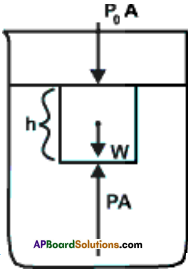1) Let us consider a container which contains a liquid in it of density ‘ p

2) Consider cylindrical column of height ‘h’ from the surface of the liquid of cross sectional area “A”.

3) The volume of the liquid column V = Ah
Mass = Volume x density
⇒ m = Ahρ
Weight W = mg = Ahρg
From Newton’s law the net force on the liquid column is zero, because it is at rest.

4) The forces acting on the water column are
a) Weight (W), vertically down
b) Force on top surface due to atmospheric pressure (P0 A) acting vertically down.
c) Force on the bottom surface of the column due to static pressure of liquid (PA), acting vertically up.

5) From Newton’s law we get

PA = P0A + W ⇒ PA = P0A + hρgA =⇒ PA = A (P0 + hρg)
∴ P = P0 + hρg
P = Pressure at depth h’ from the surface of the liquid
P0 = Atmospheric pressure
∴ The pressure inside the liquid at a constant depth is constant.

Question 4.
Calculate the pressure difference at different levels of depth in fluids.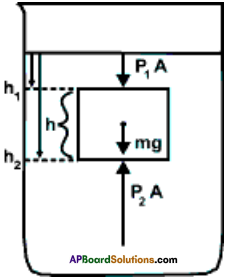1) Let us consider a cylindrical column of liquid of height ‘h’ with cross sectional area
‘A’ and let ‘P’ be the density of the liquid.

2) The pressure P1 in the liquid at depth h, is
P1 = P0 + h1ρg ………(1)

3) Similarly pressure P2 in the liquid at depth h2 is
P2 = P0 + h2ρg …………..(2)

4) From (1) and (2), P2 – P1 = h2ρg – h1pg
P2 – p1 = ρg (h2 – h1)

5) From the figure h2 – h1 = h
∴ P2 – p1 = hρg

6) The pressure difference between two levels in that liquid = hρg

7) Here ρ and g are constants, so the pressure difference increases with an increase in depth.

Question 5.
State Pascal’s principle and explain it with an example.
(OR)
Write Pascal’s law. Draw the diagram of any device works based as Pascals’ law.
Pascal’s principle :
Pascal’s principle states that external pressure applied to a enclosed body of fluid is transmitted equally in all directions throughout the fluid volume and the walls of the containing vessel.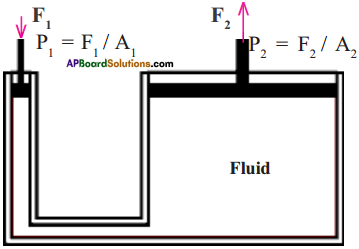Explanation :

1. Look at the figure.
2. Here we have an enclosed volume of fluid in a U-shaped tube.
3. The fluid is enclosed in the tube by two leak-proof pistons in each arm.
4. The ratio of cross-section areas of the right and left tubes is A2 : A1 and A2 > A1
5. When a force F1 is applied to the left piston, the excess pressure acting on the fluid volume is F1/A1.
6. According to Pascal’s principle, this excess pressure is transmitted equally throughout the fluid volume.
7. The excess pressure in the right-side tube (Area A2) is also F1/A1 and since its area is A2, the upward force acting on the right piston is F2 = A2 × F1/A1, which is much larger in magnitude than F1
8. Thus the application of Pascal’s principle results in a large upward force on the right piston when a small downward force is applied on the left piston.Question 6.
The volume of a solid of mass 500g is 350 cm³.
a) What will be the density of this solid?
b) What will be the relative density of the solid?
c) Will it float or sink in water?